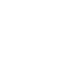# Mar 27 ACT & SAT Question of the Day

If you are reading this in an email you received from me, do not click the link to sat.collegeboard.org below. Use the link to my website that is farther down on the email. If you are seeing this in my blog, do the SAT Question of the Day by clicking on this link:

http://sat.collegeboard.org/practice/sat-question-of-the-day?src=R&questionId=20130327 (This link takes you to today’s question. If you use my archive, you will see the question related to my SAT explanation for that date.)

The answer is D.  There are two easy steps to answering Sentence Completion Questions.  First, imagine the sentence as the topic sentence of a paragraph.  Second, ask yourself, “What would you predict the paragraph would tell us?  This sentence tells us that some historians “revile” (which is negative) Livingstone “while others” (which signals a change in direction) see him as positive.  Those key words and phrases tell us his reputation was mixed, both positive and negative.  Looking at the words for the first blank, we only find one word that means his reputation was both positive and negative: dichotomous.  Checking the second word that goes with dichotomous, supports our answer since it is a positive word.  We’re all done.

What would happen if you had not known the word dichotomous?   You could look at the second column of answers and picked only positive words and kept words you didn’t know.  For example, you know praise is positive and maybe you had to keep exalt since you didn’t know what it meant.  You probably know substantial means “a large amount of something.” Since the topic of the sentence isn’t about an amount, you could eliminate A.  Again, that would leave you with D.

Always keep my strategy of treating the sentence as a topic sentence and predicting words in mind.  It will focus you on the right answers since they will always be words that are consistent with the topic of the sentence..

Let’s take a look at the ACT question.

http://www.act.org/qotd/ (The ACT staff does not put a date on their questions so if you click on an archived blog, you’ll get today’s question and the old explanation. Sorry. The SAT staff has dated their questions; so, the archive is helpful. The ACT folks simply don’t do that.)

The answer is D.  This is a pretty common math fact that shows up on the ACT and you need to be sure to recognize it.  The equation is the graph for a circle on a coordinate grid.  (x-2) tells us the center of the circle has an x-coordinate of +2.  The (y-4) tells us the y-coordinate for the center of the circle is 4.  Be sure to note the change in sign from the equation to the coordinate.  Then the right side of the equation is going to tells us the square of the radius; in this case it is 9.  Since the area of a circle is πr2 and we know that r2 is 9, then the answer has to be D.

Don’t waste time doing what the ACT explanation tells you to do for this question.  They tell you to take the square of 9 which is 3 to figure out the radius and then square the radius to get 9!!  Why would you bother.  The equation for the graph of a circle already tells you the radius squared.  Just multiply it (9) times π to get the area of the circle which is the answer to this question.

Have a great day.

The Wizard